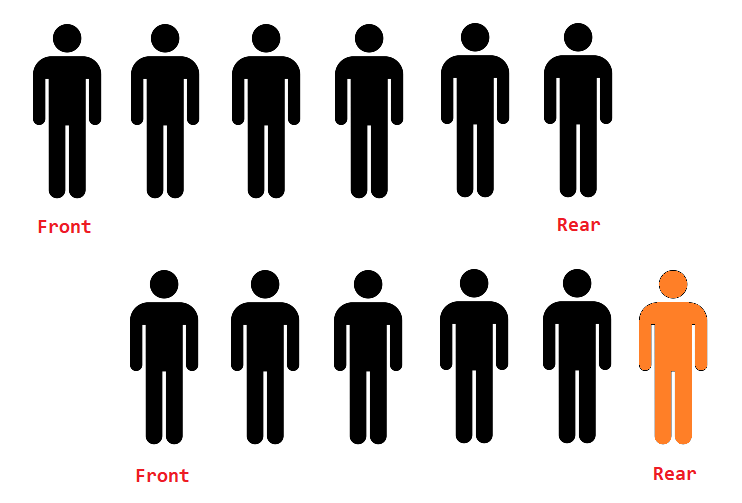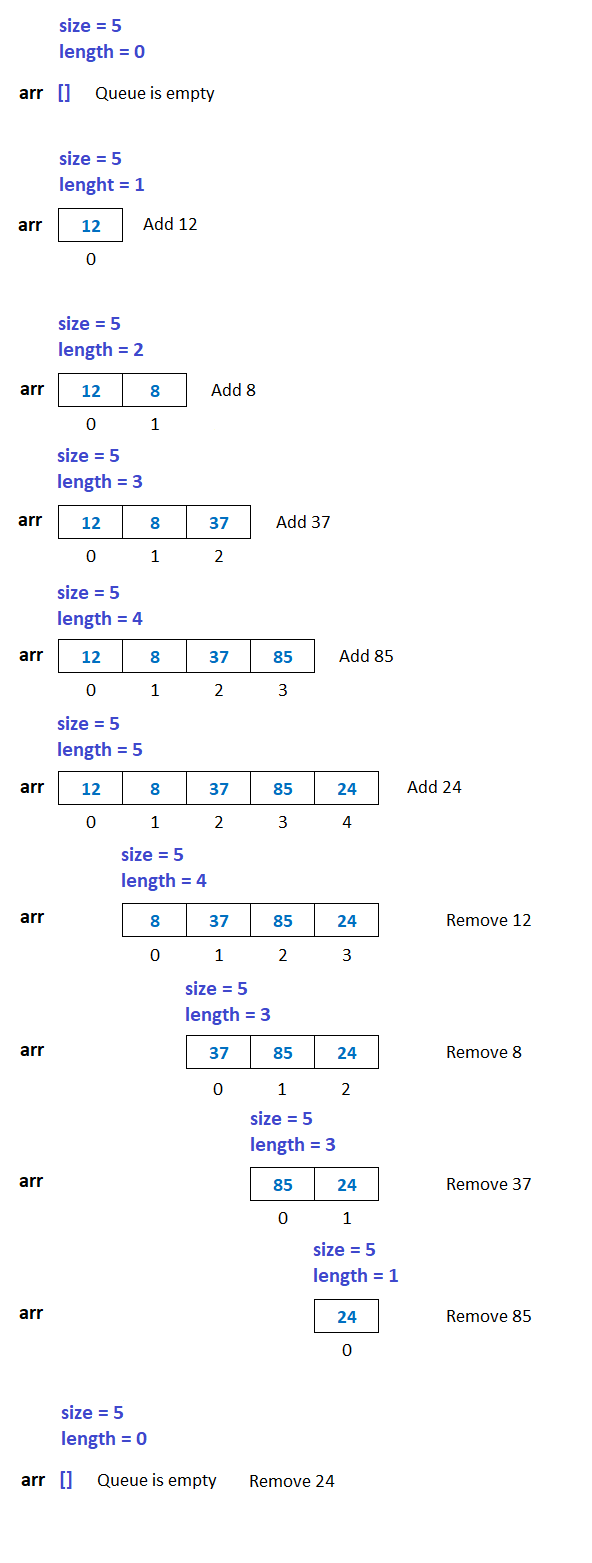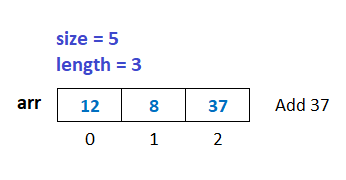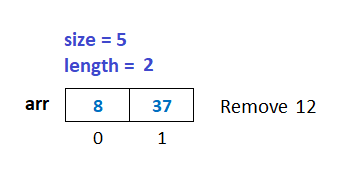# Queue in Python Programming

###### User-Defined Data Structures in Python

In this lesson, we will understand what is Queue in Python Programming and how to create them along with some examples.

## What is Queue in Python

A Queue in Python is a user-defined data structure in which we can add elements only at one end, called the rear of the queue, and delete elements only at the other end, called the front of the queue.

We can see the example of a queue in our daily life as the queue of people.In the above image, we can see that the new people can join the queue from the rear end, and when the work is over, the people at the front will leave first.## Operation on Queue

There are two operations possible on the queue.

• Delete - When we delete an element from the queue.

To understand how the above operations work on a queue. See the example given below.The above image shows that when we add a new number to the queue, the array's length is increased by 1. Similarly, when we delete a number from the queue, the array's length is decreased by 1. The array size is 5 because we want to store maximum 5 numbers in the queue.

The queue behaves in a first in, first out manner. It means that the numbers added to the queue are removed first from the queue.

So a queue is also known as FIFO (First In First Out) data structure.

## Implementation of Queue in Python

The queue in python programming can be implemented in two ways using:

• Array or List

In this lesson, we will see the implementation of a queue using an array. We will discuss how to implement queue using a singly linked list later in the subsequent lesson.

### Add Operation on Queue using Array

Suppose, we want to store 5 numbers in a queue. In that case the maximum size of the array will be 5.

For add operation on the queue first, we check if the array's length is equal to the size of the array. Then, we will display a message Queue is full. Else we will append the number in the array.

#### Example

``````size = 5
arr = array('i',[])

if len(arr)==size:
print('Queue is full')
else:
n=int(input('Enter a number '))
arr.append(n)``````

If we insert three numbers, say 12, 8, and 37, into the queue, the queue will look as shown in the image below.### Delete Operation on Queue using Array

For the delete operation on the queue, first, we check if the array's length is equal to 0. Then, we will display a message Queue is empty. Otherwise, we will remove the number stored at index 0 from the array using the pop(0) function.

#### Example

``````if len(arr)==0:
print('Queue is empty')
else:
print('Number removed = %d' %(arr.pop(0)))``````

If we delete the number 12 from the queue, the queue will look as shown in the image below.## Program of Queue using Array

Below is the complete program of the queue in python using an array of size 5.

#### Example

``````from array import *
import os

size = 5    # Maximum numbers to be stored in the array. We can increase the quantity by changing the value 5 with a new one
arr = array('i',[])     # An empty array for queue implementation

while 1:    # An infinite while loop
os.system('cls')    # Clear the console screen in Windows OS. For Linux and MacOS use os.system('clear')
print('2. Delete')
print('3. Display')

if ch==1:
# Check if the queue is full
if len(arr)==size:
print('Queue is full')
input('Press enter to continue...')     # Pause the loop so that the user can see the above message Queue is full
else:
# If queue is not full then append the number in the array
n=int(input('Enter a number '))
arr.append(n)

elif ch==2:
# Check if the queue is empty
if len(arr)==0:
print('Queue is empty')
input('Press enter to continue...')     # Pause the loop so that the user can see the above message Queue is empty
else:
# If queue is not empty then remove the number at index 0 from the array
print('Number Removed = %d' %(arr.pop(0)))
input('Press enter to continue...')     # Pause the loop so that the user can see the above message Number Removed = ?

elif ch==3:
# Check if the queue is empty
if len(arr)==0:
print('Queue is empty')
input('Press enter to continue...')     # Pause the loop so that the user can see the above message Queue is empty
else:
# if queue is not empty then display all the number
for i in range(0,len(arr)):
print(arr[i], end=' ')
input('\nPress enter to continue...')     # Pause the loop so that the user can see full queue on the screen
else:
print('Invalid Choice')
input('Press enter to continue...')     # Pause the loop so that the user can see the above message Invalid Choice``````